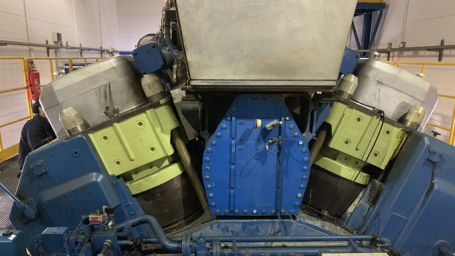Interactive

Nuclear Power Principles and Design

Martech
Updated Jan 21, 2021

After this lesson, you will be able to explain the basic theory and operation of a nuclear power plant. You will also be able to explain the basic process of nuclear fission and describe various nuclear reactor designs. • Describe the type of fuel used in nuclear power plants • Explain the purpose of control rods in nuclear reactors • Describe the use of coolants in nuclear reactors • Explain the use of moderators in nuclear reactors • Explain the process of nuclear fission • Describe the basic design of a pressurized water reactor • Describe the basic design of a boiling water reactor • Describe the basic design of heavy water reactors • Describe the basic design of gas cooled reactors • Describe the basic design of liquid metal fast breeder reactors • Describe the basic desig